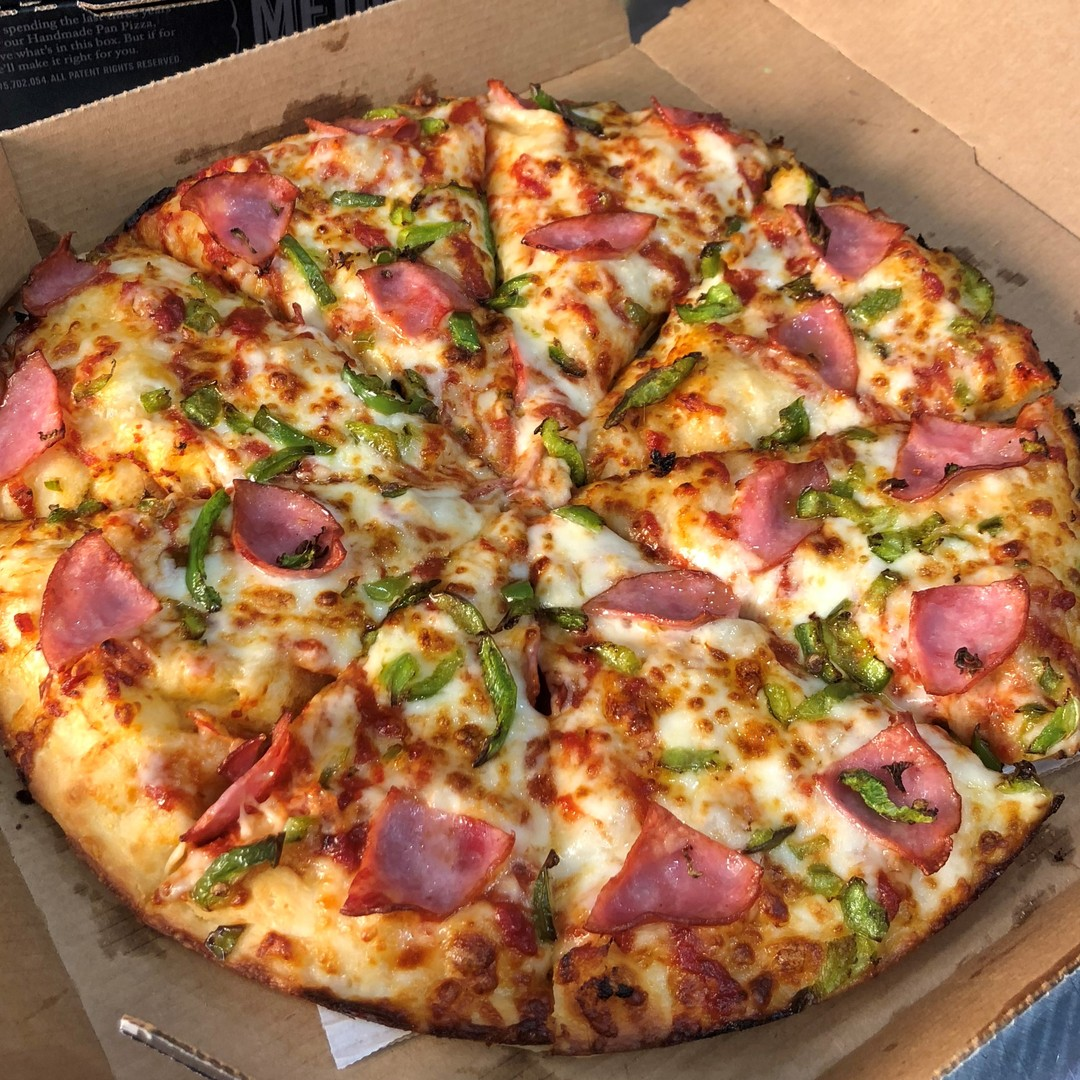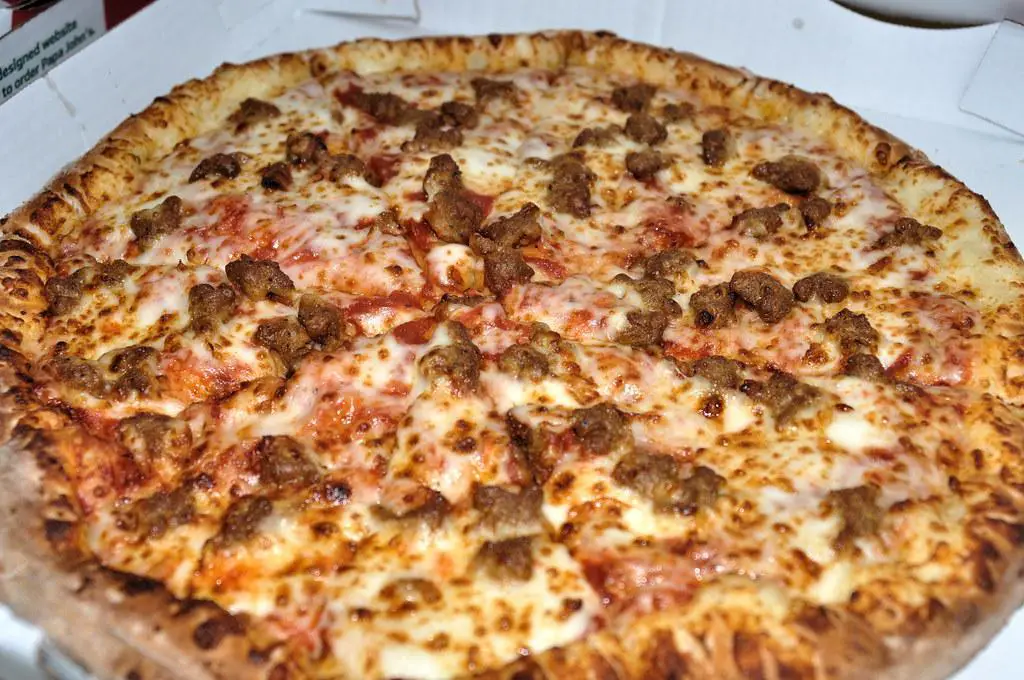# What’s the Better Deal? 2 Mediums or 1 Large Pizza?

What’s the better deal? 2 mediums or 1 large pizza? It is a simple yet challenging question that leaves people debating on which is the better deal.

When it comes to getting a better deal, you want to get more pizza for a lower price or at least the same price as the other option.

Sometimes, it is not about the quantity or the number of pizzas ordered that makes you have more pizza. Having more pizza also relies on the actual size in inches and the cost.

So, do you really get more pizza with 2 mediums than just 1 large pizza? If so, is it a better deal in terms of cost? If not, then is 1 large pizza a better deal then?

To answer these questions, let’s do the math.

There’s no better way to know which has a better deal than leaving it to mathematics. After all, numbers don’t lie and you can’t argue with facts.

In this article, the question is answered by computing the actual surface area of a medium and large pizza.

The value of the pizza is determined by calculating the area and the cost per area.

As a bonus, the article also shows a comparison of medium vs. large pizzas at some of the best pizza chains in the United States.

In summary, you will learn about the following topics:

• How Big Is A Medium Pizza?
• How Big Is A Large Pizza?
• How To Compute The Size Difference By Surface Area
• How To Compute The Cost Per Square Inch
• A Comparison Of Pizza Chains: Medium Vs Large Pizzas
• The Verdict: What’s the Better Deal?

## How Big Is A Medium Pizza?Photo: Dominos Medium Pizza Size

A medium pizza is 12 inches in diameter and cut into 8 slices. This is the most common size and number of slices offered in most pizza chains.

You can order this standard size from Domino’s, Papa John’s, Pizza Hut, and Marco’s Pizza, to name a few.

Most pizza chains have 12-inch pizzas but may still vary in the number of slices and crust styles offered.

For instance, medium pizzas from Casey’s Pizza are cut into 6 slices by default.

At Chuck E. Cheese, the medium pizzas are typically cut into 10 slices instead.

## How Big Is A Large Pizza?Photo: Papa Johns Large Pizza Size

A large pizza is commonly 14 inches in diameter with 8 slices.

However, some pizza chains have a different standard number of slices varying from 6, 10, and 12.

For instance, Godfather’s Pizza serves large pizzas with 10 slices, whereas Domino’s large Brooklyn Style Pizza has 6 slices.

At Costco’s, they only offer one size, which is a large 18-inch pizza with 12 slices.

You may also find 16-inch pizzas in some pizzerias. However, a 16-inch pizza is usually offered as an extra large size and is not typically called large.

## How To Compute The Size Difference By Surface Area

Now that you know the size of a medium and large pizza, let’s crunch the numbers and determine the surface area of a pie in square inches.

Surface area measures the total space occupied by an object’s surface. Knowing a pizza’s surface area is important to calculate its cost per square inch.

The cost per slice may not be accurate and reliable in determining the pizza’s value since not all pizza chains have the same number of slices.

Moreover, it will also depend on the number of slices you choose to customize.

Thus, it is better to determine which pizza gives good value for money based on the cost per square inch.

To calculate the surface area of a pizza, you will use the following values:

• π or pi (3.14)
• Diameter (pizza size in inches) or Radius (diameter divided by 2)

Using these values, the formula for calculating the surface area of a circle is as follows:

• Area = π X radius²

OR

• Area = π × (diameter/2)²

Although there are different pizza sizes in pizza chains, we will compute the surface area using the following data as a standard basis:

• Medium Pizza Size: 12 inches and 8 slices
• Large Pizza Size: 14 inches and 8 slices

Using the formula and the size of a medium and large pizza, follow these steps to determine the surface area of a medium and large pizza.

### How To Compute The Surface Area Of 2 Medium Pizzas

Area = π × (12 inches/2)²

1. First, divide 12 by 2. You will get 6 inches.
2. Then, 6² (6 X 6) is 36.
3. Multiply 36 by π or pi (3.14). You will get 113.04 in² or 113 in².
4. For 2 medium pizzas, multiply 113 in² by 2. You will get 226 in².
5. Thus, the surface area for 2 medium pizzas is 226 in².

### How To Compute The Surface Area Of 1 Large Pizza

Area = π × (14 inches/2)²

1. First, divide 14 by 2. You will get 7 inches.
2. Then, 7² (7 X 7) is 49.
3. Multiply 49 by π or pi (3.14). You will get 153.86 in² or rounded up to 154 in².
4. Thus, the surface area for 1 large pizza is 154 in².

#### Table Of Surface Area Of 2 Medium Pizzas And 1 Large Pizza

 Pizza Pizza Size Surface Area 2 Medium Pizzas 12 inches X 2 113 in² X 2 = 226 in² 1 Large Pizza 14 inches 154 in²

## How To Compute The Cost Per Square Inch

You now know that 2 medium pizzas are larger in size than 1 large pizza. But is it better in value?

Will it be cheaper to get 2 medium pizzas than 1 large pizza?

Let’s use Domino’s classic pepperoni style pizza in Hand Tossed crust as an example.

Domino’s Ultimate Pepperoni Pizza in Hand Tossed crust for a medium size costs \$15.99, while a large size costs \$18.99.

If you are to compute the costs, it would be:

• 2 Medium Pizzas: \$15.99 X 2 = \$31.98
• 1 Large Pizza: \$18.99

Naturally, 2 medium pizzas would cost more than 1 large pizza if you are to compute by price.

Since you want to know which has a better value, you can determine that by calculating the price per square inch instead.

To calculate the price per square inch, you need the following values:

• Total Cost of the pizza
• Surface Area of the pizza

With these values, you will divide the total cost of pizza by the Surface Area of the pizza. The formula to use is:

• Cost per square inch = Total Cost / Surface Area

### How To Compute The Cost Per Square Inch Of 2 Medium Pizzas

Cost per square inch = \$31.98 / 226 in²

1. \$31.98 divided by 226 in² =  \$0.1416 per square inch
2. Thus, 2 medium pizzas cost about 14 cents per square inch.

### How To Compute The Cost Per Square Inch Of 1 Large Pizza

Cost per square inch = \$18.99 / 154 in²

1. \$18.99 divided by 154 in² =  \$0.1233 per square inch
2. Thus, 1 large pizza costs about 12 cents per square inch.

#### Table Of Cost Comparison Of 2 Medium Pizzas Vs 1 Large Pizza

 Pizza Size in inches Price Surface Area in in² Cost per in² 2 Medium Pizzas 12 inches X 2 \$15.99 X 2 = \$31.98 113 in² X 2 = 226 in² 14 cents per square inch 1 Large Pizza 14 inches \$18.99 154 in² 12 cents per square inch

Based on the results, the cost per square inch of 1 large pizza is generally cheaper than 2 medium pizzas by 2 cents.

Although it is a minimal difference, at least you can see which has a higher cost per square inch.

## A Comparison Of Pizza Chains: Medium Vs Large Pizzas

Let’s compare the differences of medium and large pizzas at top pizza chains in the U.S.

The table shows a quick comparison of the price, size, surface area, and cost per square inch of both medium and large pizzas.

Take note that the prices listed below may vary per store location, so it is best to check with your local store for updated prices.

#### Table Of Comparison Of Pizza Chains: Medium Vs Large Pizzas

 Pizza Chain Medium Large Price Size in inches Surface Area in in² Cost per in² Price Size in inches Surface Area in in² Cost per in² Pizza Hut Medium Vs Large \$13.49 12 inches 113 in² 11 cents per square inch \$15.49 14 inches 154 in² 10 cents per square inch Papa Johns Medium Vs Large \$11.09 12 inches 113 in² 9 cents per square inch \$14.09 14 inches 154 in² 9 cents per square inch Dominos Medium Vs Large \$15.99 12 inches 113 in² 14 cents per square inch \$18.99 14 inches 154 in² 12 cents per square inch Jet’s Pizza Medium Vs Large \$12.49 12 inches 113 in² 11 cents per square inch \$15.49 14 inches 154 in² 10 cents per square inch Marco’s Pizza Medium Vs Large \$15.99 12 inches 113 in² 14 cents per square inch \$18.99 14 inches 154 in² 12 cents per square inch Godfather’s Pizza Medium Vs Large \$23.24 12 inches 113 in² 20 cents per square inch \$26.74 14 inches 154 in² 17 cents per square inch Casey’s Pizza Medium Vs Large \$12.99 12 inches 113 in² 11 cents per square inch \$13.99 14 inches 154 in² 9 cents per square inch Papa Murphy’s Medium Vs Large \$9.99 12 inches 113 in² 8 cents per square inch \$11.99 14 inches 154 in² 7 cents per square inch Hungry Howie’s Pizza Medium Vs Large \$10.99 12 inches 113 in² 9 cents per square inch \$12.99 14 inches 154 in² 8 cents per square inch Chuck E. Cheese Medium Vs Large \$18.99 12 inches 113 in² 16 cents per square inch \$21.99 14 inches 154 in² 14 cents per square inch

## The Verdict: What’s the Better Deal?

Which is the better deal when it comes to getting more pizzas and a cheaper cost per square inch?

If you want more pizzas, then it is obviously 2 medium pizzas. 2 medium pizzas have a larger surface area than 1 large pizza, with a total area of 226 in².

There is no doubt that you will get the most of your money with 1 large pizza since it is cheaper, with a cost per square inch of 12 cents per square inch.

• More pizza in surface area = Choose 2 medium pizzas
• Cheaper cost per square inch = Choose 1 large pizza

Some might say that 1 large pizza is a better deal. However, that is because they compared it to an 18-inch large.

If you are to use the formula above and compare, you will get the following results for an 18-inch pizza:

Area = π × (18 inches/2)²

• 18-inch pizza Surface Area = 254.34 in² or 254 in²

Since the surface area of an 18-inch pizza is 254 in², it is larger and a better deal in cost than 2 medium pizzas.

So if you can find a pizza place with 18-inch pizzas, you can opt for that instead if the cost for 1 18-inch pizza is cheaper than 2 medium pizzas.

## What Do You Think?

What do you think about the verdict? Do you agree or disagree with the results? Share your thoughts in the comments section below.